#### How to calculate Geometric Mean?

In this Excel tutorial you will teach yourself how to calculate geometric mean in Excel.

## What is geometric mean?

The means in statistical surveys are classified as measures of the central tendency. You could say that they indicate in some way the “center” of the data set. Since “center” is a vague concept, means can also be defined in various ways. Examples of the means we already know are the median and the arithmetic mean.

The geometric average is used to characterize the similarities of statistical communities due to the distinguished feature. All values of the series are used for the measurement. This allows for an average measurable feature in a given set. The advantage of the geometric mean is that it is less responsive to extreme values (sometimes random values) than the arithmetic mean.

The geometric mean is used in the case of values that change exponentially (e.g. in demography, when calculating the average rate of growth of the national income, in calculations related to the geometric sequence). We use the geometric mean to calculate the average growth rate – of course, if the statistical data inform about the average increases of the analyzed value in relation to the previous year (period).

Note: Geometric mean is always lower than arithmetic mean.

## Geomean function

To calculate a geometric mean in Excel you need to know how to use GEOMEAN function.

### Geomean syntax

=GEOMEAN (number1, [number2], …)

• number1 is a first value to calculate. It can be a number, reference or a range.
• number2 is optional argument to provide more values for calculations. Up to 255 arguments can be provided.

Arguments cannot be less than 0. When any number is less than 0, GEOMEAN function will return #NUM! error.

When text value will be provided as a argument the function will return #VALUE! error.

## How to calculate geometric mean in Excel?

Let’s see example data of portfolio investment.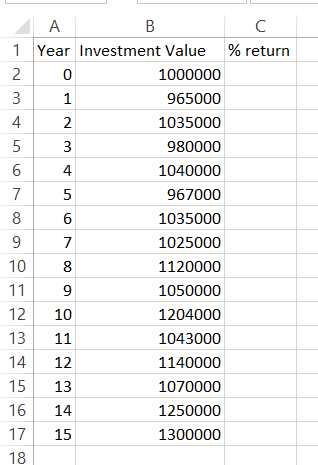We know percent return every year. So it is easy to calculate return on investment.

To calculate it you need to divide final value by starting value. -1 is just to get percentage of the change. Excel formula is =B3/B2-1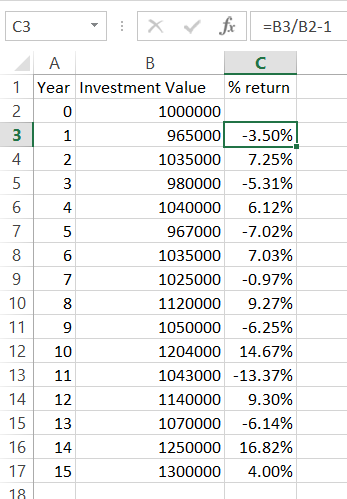Let’s first calculate arithmetic average using Excel average function.

Excel formula this time is =AVERAGE(C13:C17)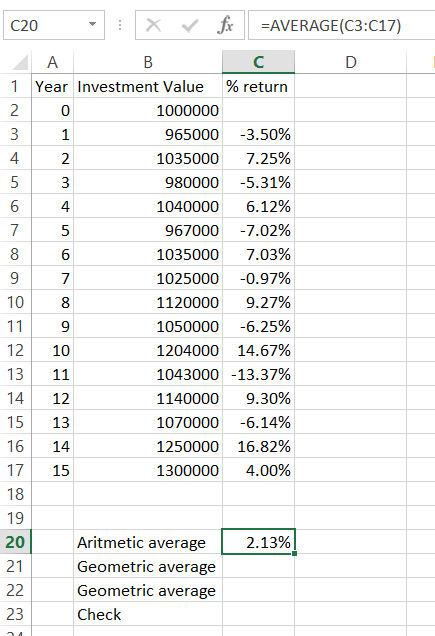## Calculating Geometric Mean by Values

First method to calculate geometric mean is to calculate values. Here’s geometric formula for this method:

Geometric Average = (Result Value / Starting Value ^ (1 / Investment Period) – 1

For this method just use Excel formula =(B17/B2)^(1/\$A\$17)-1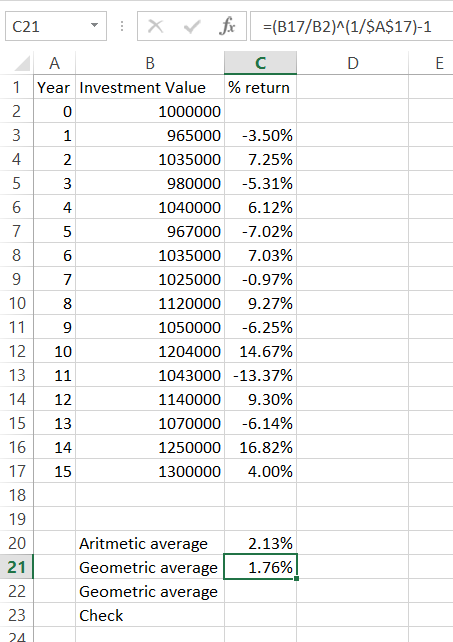## Calculating Geometric Mean by percent return

The second method to calculate geometric average is to base on return percentage.

Use Sumproduct function for that purpose.

=SUMPRODUCT(GEOMEAN(C3:C17+1)-1)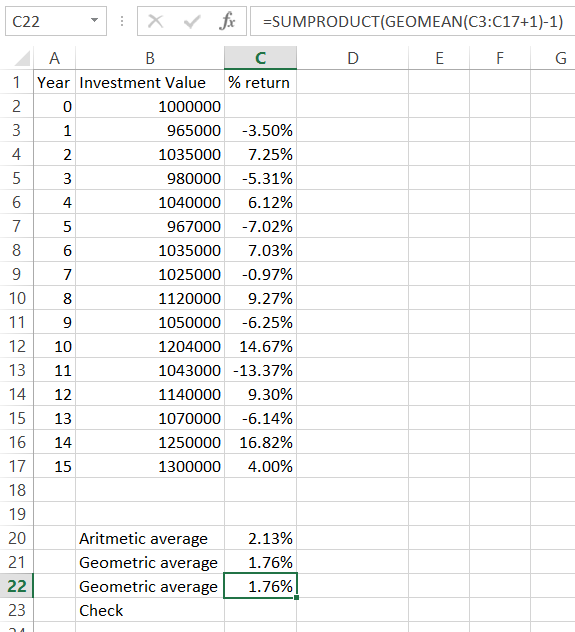To check if geometric mean calculations are proper you can calculate it step by step.

To calculate that you need multiply starting value by 1 + geometric mean and power it by investment periods number.

Formula is =B2*(1+C21)^15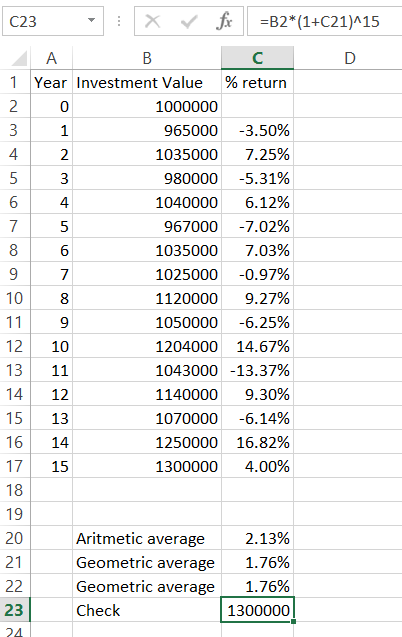Results are correct. Geometric average calculator worked fine. You can download it here.